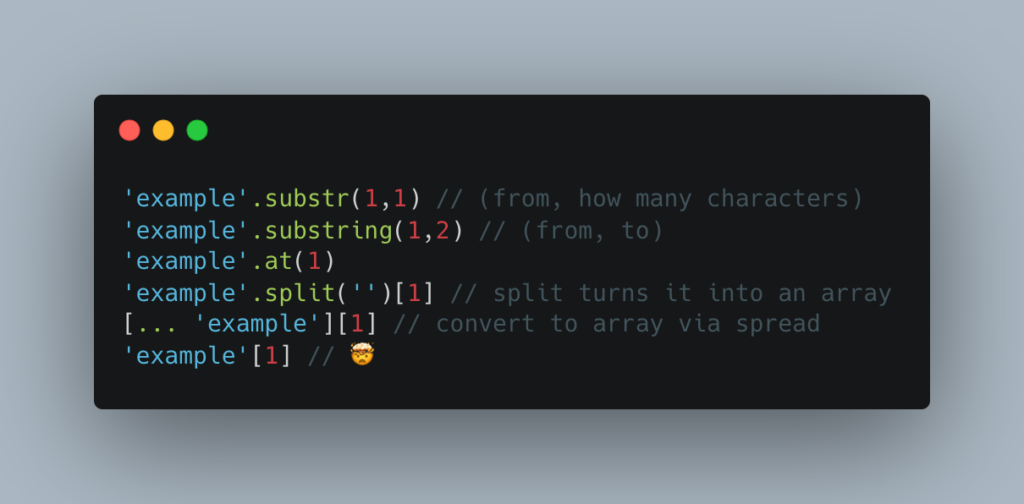Christian Heilmann

# The many ways to select the n-th character from a string.

Friday, June 2nd, 2023 at 10:20 amA question I came across the other day during a JavaScript application test was this:

How would you select the n-th character from the word “Example”?

The fun thing here is that there are lots of different ways to do that. The one I always forget about is that you can access the index of a string directly. This works in JavaScript and PHP!

```'example'.substr(1,1) // (from, how many characters) 'example'.substring(1,2) // (from, to) 'example'.at(1) 'example'.split('') // split turns it into an array [... 'example'] // convert to array via spread 'example' // &#x1f92f;```

Now, when checking that a string is a certain length, you normally use the length property, but you could also simply check if the index exists, to make it shorter.

```let str = 'example'; let amount = 4; if (str.length > amount) { console.log('string is long enough'); } if (str[amount + 1]) { console.log('string is long enough'); }```

The question is if this performs better or not. Also, the length bit might make it more readable.

Other problems are that zero-indexing can be confusing (hence the `amount+1`) and that when you use the index you don’t get a boolean returned but instead the character or `undefined`. So if you wanted to write this as a function you need to write something akin to:

`const isXlong = (str, y) => str[y + 1] ? true : false;`

Which makes it less readable again.